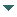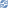# gvSIG

##### Personal tools
You are here: Home Surface
gvSIG DesktopgvSIG Desktop

Cached time 11/21/13 11:24:39# Surface

Up one level
 ◄ Previous Arc Next: Circle ►

To create a surface we can use the generic method create from the manager. To establish the coordinate's values, we can use a GeneralPathX type object. The steps to create a triangular polygon on the coordinates 0,0 0,5 5,5 can be the next:

```Surface surface = (Surface)geometryManager.create(TYPES.SURFACE, SUBTYPES.GEOM2D);
GeneralPathX generalPathX = new GeneralPathX();
generalPathX.moveTo(0, 0);
generalPathX.lineTo(0, 5);
generalPathX.lineTo(5, 5);
surface.setGeneralPath(generalPathX);
```

Like it happens on the Curve, a Surface must be breated from the own model's objects. In the polygon case, they will be Point objects. The next code let us to create a polygon with the same coordinates like the previous example:

```Point point1 = geometryManager.createPoint(0, 0, SUBTYPES.GEOM2D);
Point point2 = geometryManager.createPoint(0, 5, SUBTYPES.GEOM2D);
Point point3 = geometryManager.createPoint(5, 5, SUBTYPES.GEOM2D);
Surface surface = (Surface)geometryManager.create(TYPES.SURFACE, SUBTYPES.GEOM2D);
```

The addVertex method inserts a new vertex in the last position in a Surface object and it should be used in the geometry creation. In the other hand, there're more methods which let us the edition of the internal vertex's coordenates that compose the polygon. For example, if we want to change the final point of the polygon in the last example, we could do this:

```Point endPoint = geometryManager.createPoint(0, 10, SUBTYPES.GEOM2D);
surface.setVertex(2, endPoint);
```

The setVertex method fixes the value of one of the internal points which are part of the polygon composition and replace it for the point which was previously. The first parameter is the internal point position that is necessary to modify.

In the other hand, we can also insert a point inside the polygon. For example, to insert the point 0,7 in the third position we must execute the following code:

```Point middlePoint = geometryManager.createPoint(0, 7, SUBTYPES.GEOM2D);
surface.insertVertex(2, middlePoint);
```

The first parameter is the point position that we want to put in. In this case, the resultant polygon will have the coordinates 0,0 0,5 0,7 and 0,10 such as we have been defining.

 ◄ Previous Arc Next: Circle ►